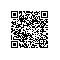# js变量以及其作用域详解

Javascript和Java、C这些语言不同，它是一种无类型、弱检测的语言。它对变量的定义并不需要声明变量类型，我们只要通过赋值的形式，可以将各种类型的数据赋值给同一个变量。例如：

i=100;//Number类型
i="variable";//String类型
i={x:4};//Object类型
i=[1,2,3];//Array类型

JS的这种特性虽然让我们的编码更加灵活，但也带来了一个弊端，不利于Debug，编译器的弱检测让我们维护冗长的代码时相当痛苦。

JS中变量申明分显式申明隐式申明

var i=100;//显式申明

i=100;//隐式申明

三、全局变量和局部变量

<SCRIPT LANGUAGE="JavaScript" type="text/javascript">
//定义一个输出函数
function outPut(s){
document.writeln(s)

//全局变量
var i=0;
//定义外部函数
function outer(){
//访问全局变量
outPut(i); // 0
//定义一个类部函数
function inner(){
//定义局部变量
var i = 1;
// i=1; 如果用隐式申明　那么就覆盖了全局变量i
outPut(i); //1

inner();
outPut(i); //0

outer();
</SCRIPT>

<SCRIPT LANGUAGE="JavaScript" type="text/javascript">
//定义一个输出函数
function outPut(s){
document.writeln(s)

//全局变量
var i=0;
//定义外部函数
function outer(){
//访问全局变量
outPut(i); // 0
//定义一个类部函数
function inner(){
outPut(i); //undefiend
var i=1;
outPut(i); //1

inner();
outPut(i); //0

outer();
</SCRIPT>

function inner(){
var i; //定义但不赋值
outPut(i); //undefiend
i=1;
outPut(i); //1

JS不同于JAVA、C这些语言，在变量申明时并不需要声明变量的存储空间。变量中所存储的数据可以分为两类：基本类型和引用类型。其中数值、布尔值、null和undefined属于基本类型，对象、数组和函数属于引用类型。

<SCRIPT LANGUAGE="JavaScript" type="text/javascript">
//定义一个输出函数
function outPut(s){
document.writeln(s)

var a = 3;
var b = a;
outPut(b);
//3
a = 4;
outPut(a);
//4
outPut(b);
//3
</SCRIPT>

<SCRIPT LANGUAGE="JavaScript" type="text/javascript">
//定义一个输出函数
function outPut(s){
document.writeln(s)

var a_array = [1,2,3];
var b_array = a_array;
outPut(b_array); //1,2,3
a_array = 4;
outPut(b_array);//1,2,3,4
</SCRIPT>

上面是对引用类型的变量赋值，实际上他们传递的是对内存地址的引用，因此对a_array和b_array的存取，实际上都是操作的同一块内存区域。如果希望重新分配内存空间存储引用型变量，那么我就需要使用克隆方法或者自定义方法来复制引用变量的数据。使用钉钉扫一扫加入圈子
+ 订阅